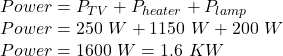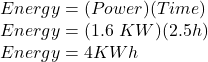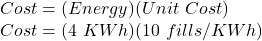## A student’s room has a TV (250 W) ,heater (1150 W) and lamp (200 W) Electricity costs 10 fills per KWh. Calculate how much it would cost to

Question

A student’s room has a TV (250 W) ,heater (1150 W) and lamp (200 W) Electricity costs 10 fills per KWh. Calculate how much it would cost to run these three appliances for a total of 2 hour 30 minutes

in progress 0
5 months 2021-08-21T01:39:44+00:00 1 Answers 5 views 0

Cost = 40 fills

Explanation:

First, we will calculate the total power consumed by all appliances:Now, we will calculate the energy required:Now, for the cost we use the following formula:Cost = 40 fills